## MP Board Class 7th Maths Solutions Chapter 4 Simple Equations Ex 4.3

Question 1.
Solve the following equations:
(a) $$2 y+\frac{5}{2}=\frac{37}{2}$$
(b) 5t + 28 = 10
(c) $$\frac{a}{5}+3$$ = 2
(d) $$\frac{q}{4}+7$$ = 5
(e) $$\frac{5}{2} x$$ = -10
(f) $$\frac{5}{2} x=\frac{25}{4}$$
(g) $$7 m+\frac{19}{2}$$ = 13
(h) 6z + 10 = -2
(i) $$\frac{3 l}{2}=\frac{2}{3}$$
(j) $$\frac{2 b}{3}-5$$ = 3
Solution: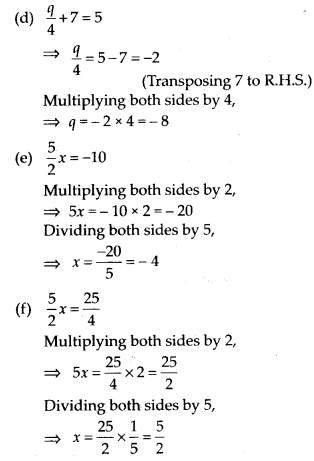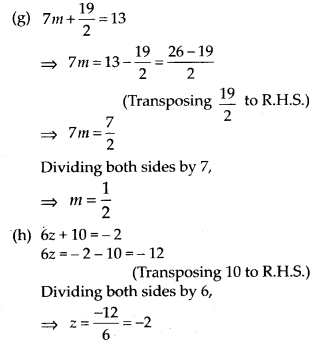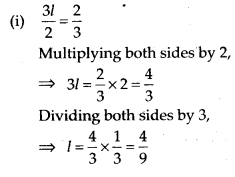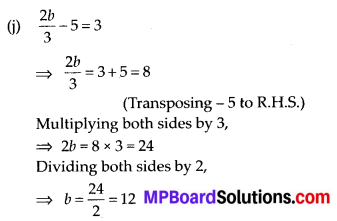Question 2.
Solve the following equations.
(a) 2(x + 4) = 12
(b) 3(n – 5) = 21
(c) 3(n – 5) = -21
(d) -4(2 + x) = 8
(e) 4(2 – x) = 8
Solution:
(a) 2(x + 4) = 12
Dividing both sides by 2,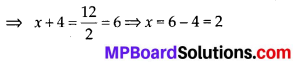(Transposing 4 to R.H.S.)

(b) 3(n – 5) = 21
Dividing both sides by 3,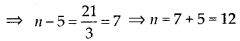(Transposing – 5 to R.H.S.)

(c) 3(n – 5) = -21
Dividing both sides by 3,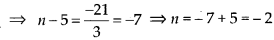(Transposing – 5 to R.H.S.)

(d) – 4(2 + x) = 8
Dividing both sides by – 4,(Transposing 2 to R.H.S.)

(e) 4(2 – x) = 8
Dividing both sides by 4,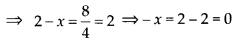(Transposing 2 to R.H.S.)
⇒ x = 0Question 3.
Solve the following equations:
(a) 4 = 5(p – 2)
(b) -4 = 5(p – 2)
(c) 16 = 4 + 3(t + 2)
(d) 4 + 5(p – 1) = 34
(e) 0 = 16 + 4(m – 6)
Solution:
(a) 4 = 5(p – 2)
Dividing both sides by 5,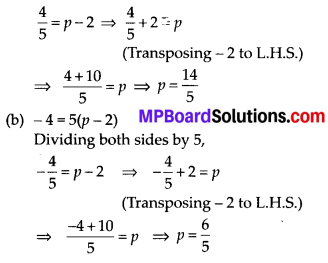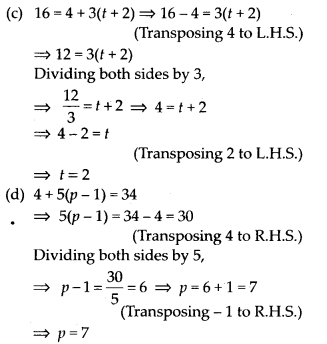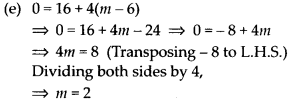Question 4.
(a) Construct 3 equations starting with x= 2.
(b) Construct 3 equations starting with x = -2.
Solution:
(a) x = 2
Multiplying both sides by 5,
5x = 10 … (i)
(b) Let the number be x.
Subtracting 3 from both sides,
5x – 3 = 10 – 3
5x – 3 = 7 …(ii)
Dividing both sides by 2,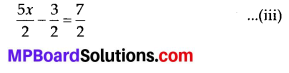(b) x= -2
Subtracting 2 from both sides,
x – 2 = -2 – 2 ⇒ x – 2 = -4 … (i)
Again, take x = – 2
Multiplying both sides by 6,
6 × x = -2 × 6 ⇒ 6x = -12
Subtracting 12 from both sides,
6x – 12 = – 12 – 12 ⇒ 6x – 12 = – 24 …(ii)